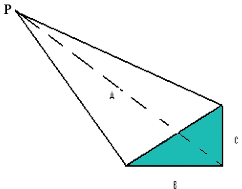SEARCH HOMEMath Central Quandaries & QueriesHello, Right now I am stuck and I feel embarrassed because I feel like the answer is so easy I should know it. I am working on a project and need to find a volume of gravel it will take to occupy this triangular prism like area. I am not sure what formulas I should use whether it be that for the volume of a pyramid or something more complex? Basically it forms a right triangle at one side then from there all points slope to one singular point about 10412mm away. I am attaching a picture drawn up in paint with the actual dimensions to clear up any confusion. Thank you for any help. CaseyHi Casey,

Here is the diagram you sent. I labeled one vertex $P$ and shaded one face. You also gave us that $A = 10412.98 \mbox{mm, } B = 1955.8 \mbox{ mm, and } C = 227 \mbox{ mm.}$This shape is called a triangular pyramid and the volume of a triangular pyramid is

$\frac13 \times \mbox{ the area of the base } \times \mbox{ the height.}$

In your situation I would take the face I shaded green as the base since its area is easy to calculate. The area of the base is

$\frac12 B \times C.$

The height is the perpendicular distance from the base to the vertex $P.$ I can't tell from your diagram if $A$ is the height, but if it is not you will need a way to find the height.

I hope this helps,
PennyMath Central is supported by the University of Regina and The Pacific Institute for the Mathematical Sciences.